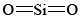# Silicon Dioxide in Earth's Crust

$$\newcommand{\vecs}{\overset { \rightharpoonup} {\mathbf{#1}} }$$ $$\newcommand{\vecd}{\overset{-\!-\!\rightharpoonup}{\vphantom{a}\smash {#1}}}$$$$\newcommand{\id}{\mathrm{id}}$$ $$\newcommand{\Span}{\mathrm{span}}$$ $$\newcommand{\kernel}{\mathrm{null}\,}$$ $$\newcommand{\range}{\mathrm{range}\,}$$ $$\newcommand{\RealPart}{\mathrm{Re}}$$ $$\newcommand{\ImaginaryPart}{\mathrm{Im}}$$ $$\newcommand{\Argument}{\mathrm{Arg}}$$ $$\newcommand{\norm}{\| #1 \|}$$ $$\newcommand{\inner}{\langle #1, #2 \rangle}$$ $$\newcommand{\Span}{\mathrm{span}}$$ $$\newcommand{\id}{\mathrm{id}}$$ $$\newcommand{\Span}{\mathrm{span}}$$ $$\newcommand{\kernel}{\mathrm{null}\,}$$ $$\newcommand{\range}{\mathrm{range}\,}$$ $$\newcommand{\RealPart}{\mathrm{Re}}$$ $$\newcommand{\ImaginaryPart}{\mathrm{Im}}$$ $$\newcommand{\Argument}{\mathrm{Arg}}$$ $$\newcommand{\norm}{\| #1 \|}$$ $$\newcommand{\inner}{\langle #1, #2 \rangle}$$ $$\newcommand{\Span}{\mathrm{span}}$$$$\newcommand{\AA}{\unicode[.8,0]{x212B}}$$

SiO2 is an important macromolecular solid in the earth's crust. While silicon and carbon are both Group IVA elements, silicon is reluctant to form multiple bonds, and so discretemolecules, analogous to, do not occur. In order to satisfy silicon’s valence of 4 and oxygen’s valence of 2, each silicon must be surrounded by four oxygen atoms and each oxygen atom by two silicon atoms. This general structure can be shown by Lewis diagram:Figure $$\PageIndex{1}$$ Lewis diagram representation of a silica.

About 12 percent of all the material in the crust of the earth (a layer which extends about 30 to 40 km below the surface) is silica. Silica can exist in six different crystalline forms. The best known of these is quartz. A group of substances which are closely related to silica, the feldspars, are even more important. They constitute about 50 percent of the earth’s crust. One of the simplest of the feldspars, and also one of the most abundant, is orthoclase, which has the formula KAlSi3O8. The structure of this mineral is best described in terms of the schematic Lewis diagram.Figure $$\PageIndex{2}$$ Lewis diagram of orthoclase, a feldspar.

As with silica, there is a three-dimensional network of interconnected tetrahedrons of O atoms. In orthoclase, though, every fourth tetrahedron has an Al rather than an Si atom at its center. Since Al has one less valence electron than Si, an extra electron is needed to preserve the octet structure of the network. This electron can be donated by a K atom, which then becomes a potassium ion, K+. Thus for every Al atom in the middle of a tetrahedron of O atoms, there is a K+ ion close by. The three-dimensional framework of Al, Si, and O atoms is thus a giant polyatomic ion with the formula (AlSi3O8)n. (The number of Al atoms in the crystal is n.) The crystal is electrically neutral because of the presence of nK+ ions. The actual network of tetrahedrons into which the K+ ions fit is somewhat different from that shown for quartz because the channels in the quartz network are not large enough to accommodate K+ ions.

The other feldspars are similar to orthoclase in general structure. All consist of a three-dimensional network of interlocked tetrahedrons of O with both Al and Si atoms at their centers. Since these networks are negatively charged, they must also accommodate positive ions. Na+, Mg2+, and Ca2+ ions are found as well as K+.

In addition to feldspars and silica, a further 20 percent of the earth’s crust also consists of silicon compounds. In these minerals the tetrahedrons of O around each Si atom are not as extensively interlinked as in quartz and the feldspars. An example is shown in the figure below.Figure $$\PageIndex{3}$$ Silicate sheet with the formula Si2O52. Sheets like this occur both in micas and in clays.

The flat sheet shown has the formula (Si2O52)n. In minerals such as mica these flat sheets are layered above one another and positive ions occupy the spaces between them. The overall effect is like a giant multilayered sandwich. The sheets in this structure can easily be separated and account for the ease with which crystals of mica cleave into plates a few tenths of a millimeter thick.

From ChemPRIME: 8.21: Silicon Dioxide